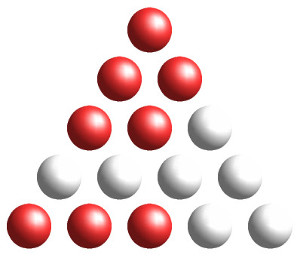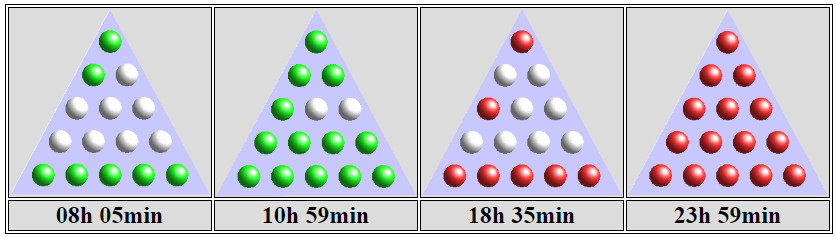# Triangular TimeAachen University physicist Jörg Pretz has devised a binary clock in the shape of a triangular array of 15 lamps. Here’s how to read it:

• When lit, the top lamp denotes 6 hours.
• Each lamp on on the second row denotes 2 hours.
• Each lamp on on the third row denotes 30 minutes.
• Each lamp on on the fourth row denotes 6 minutes.
• Each lamp on on the fifth row denotes 1 minute.

So the clock above shows 6 hours + (2 × 2 hours) + (2 × 30 minutes) + (3 × 1 minute) = 11:03. The lamps’ color, red, shows that it’s after noon, or 11:03 p.m. The same array displayed in green would mean 11:03 a.m. A few more examples:The time value assigned to each lamp is the total time value of the row below if that row contained one additional lamp.

On each row the lamps light up from left to right, so a row with n lamps can display n + 1 states (all lamps off to all lamps on). So for a triangular array with n lamps on the bottom row, the total number of states is

(n + 1) × ((n – 1) + 1) × ((n – 2) + 1) × · · · × (1 + 1) = (n + 1)!

That is, it’s a factorial of a natural number. And by a happy coincidence, the total number of minutes in 12 hours is such a factorial (720 = 6!).

“Thus the whole concept works because our system of time divisions is based on a sexagesimal system, dating back to the Babylonians, rather than a decimal system, as proposed during the French Revolution.”

There’s more info in Pretz’s article, and you can play with the clock using this applet.

(Jörg Pretz, “The Triangular Binary Clock,” Recreational Mathematics Magazine, March 2016.)